Previous: 8.3 Conservation of Mass Up: 8.3 Conservation of Mass Next: 8.3.2 Meshing Constraints

## 8.3.1 Continuity Equation

The physical quantity associated with the zeroth order moment is the carrier density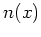: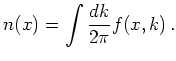The zeroth order moment equation describes the conservation of particle number, also called conservation of mass.

We derive the corresponding conservation equation by integrating the Wigner equation over all velocities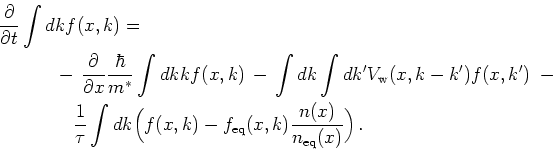(8.6)

By definition the Wigner potential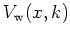is an odd function in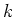. Hence the contribution from the integral(8.7)

vanishes. This property expresses conservation of particle number from potential scattering. In a similar way the term stemming from scattering also vanishes as we have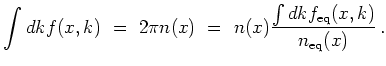With the introduction of the (particle) current density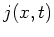: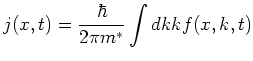(8.8)

we finally get the continuity equation (in vector notation):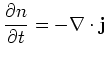The aim is to find a discretization of the full Wigner equation which conserves mass.